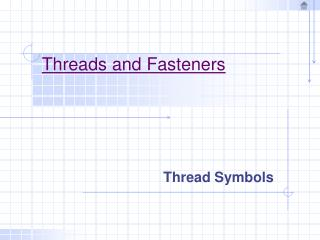# Threads and Fasteners - PowerPoint PPT PresentationDownload PresentationDownload Presentation- - - - - - - - - - - - - - - - - - - - - - - - - - - E N D - - - - - - - - - - - - - - - - - - - - - - - - - - -
##### Presentation Transcript

4. Thread Symbols Screw Thread Forms: Various forms of threads are used to hold parts together, to adjust parts with reference to each other, or to transmit power.

5. Thread Symbols Right-hand and left-hand threads: A right-hand thread is one that advances into a nut when turned clockwise (figure a). A left-hand thread is on the advances into a nut when turned counterclockwise (figure b). A thread is always considered to be right-handed unless specified otherwise. A left-handed thread is always labeled (LH) on a drawing.

6. Thread Symbols Single and Multiple Threads: A single thread is composed of one ridge and the lead is equal to the pitch (figure a). A double thread is composed of two ridges and the lead is equal to twice the pitch (figure b). A triple thread is composed to three ridges and the lead is equal three times the pitch (figure c).

7. Thread Symbols External Thread Symbols: Simplified external thread symbols are shown in figure (a) and figure (b). The threaded portions are indicated by hidden lines parallel to the axis at the approximate depth of the thread, whether in section or elevation. Schematic external thread symbols are shown in figure (c) and figure (d). Schematic threads in elevation (figure d) are indicated by alternate long and short lines at right angles to the center line. When the schematic form is shown in section (figure c) it is necessary to show the Vs. It is not necessary to show the Vs to scale. To draw the Vs use the schematic thread depth and let the pitch be determined by the 60 degree Vs.

8. Thread Symbols Internal Thread Symbols: Internal simplified and schematic thread symbols are shown below. Note that the only difference between the schematic and simplified internal thread symbols is in the section views.

9. Thread Symbols Drawing Simplified Threads Step 1: Draw the major diameter and locate the thread length of full threads. Step 2: Draw the minor diameter by marking off the thread depth (D) from the table. Step 3: From the minor diameter draw the 45 degree chamfered ends.

10. Thread Symbols Drawing Schematic Threads Step 1: Draw the major diameter and locate the thread length of full threads. Step 2: Locate the minor diameter (D) from the table and draw the 45 degree chamfered ends. Step 3: Draw lines to represent the crest of the threads spaced at a distance equal to (P) from the table. Step 4: Draw lines to represent the root of the threads centered between the crest lines to the minor diameter (D).

11. Thread Symbols Detailed Representation: Metric, Unified, and American National Threads The representation for metric, Unified, and American National Threads is the same since the flats are disregarded. The steps in drawing these threads are shown below.

12. Thread Symbols Thread Notes: The thread note for Unified and American National screw threads is shown in figure (a). The thread note for metric screw threads is shown in figure (b). Metric screw threads are designated by the letter (M) metric profile followed by the nominal size (major diameter) and the pitch, both in millimeters.